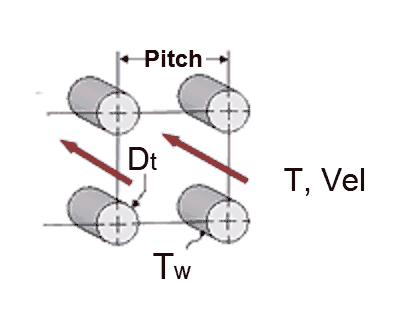Contents - Index

Tube_Bundle_NDProcedure Tube_Bundle_ND(G\$, Re, Pr, P\D, wtc: f, Nusselt) returns the average friction factor and Nusselt number for ideal longitudinal flow in a tube bundle.  The correlations are applicable to flow up, down, or horizontal.

Inputs:

G\$ - a string or string variable that is set to either 'Square' or 'Triangular', referring to the arrangement of the tubes in the tube bundle

Re - Reynolds number based on the tube diameter as the significant length with properties evaluated at the bulk average temperature

Pr - Prandtl number of the fluid evaluated at the bulk average temperature

P\D - ratio of the distance between tube centers to the tube diameter.  The correlations are applicable for 1.15 < P\D < 2.

wtc - wall temperature correction factor.  According to Taborek this parameter is determined as follows;

for gases, wtc = (Bulk temperature [K]/Wall temperature [K])^n where n=0 for cooling and n=0.55 for heating

for liquids, wtc = (Pr evaluated at bulk temperature / Pr evaluated at wall temperature)^0.14

Outputs:

f -  average friction factor [-]

Nusselt - average Nusselt number

Notes

This procedure  is called by  Tube_Bundle

The procedure provides the correlations provided by Taborek.  It will determine if the flow is laminar or turbulent and apply the appropriate correlation based on the correlation for the transitional Reynolds number provided by El-Genk et al.

Longitudinal flow can be classified as either internal or external flow.

Example:

\$UnitSystem SI C kPa kJ mass deg

G\$='SQUARE'

Re=3000

Pr=0.7

P\D=1.5

wtc=(400/450)^0.4

Call tube_bundle_nd(G\$, Re, Pr, P\D, wtc: f, Nusselt)

{Solution:

f=0.04859

Nusselt=12.91 }